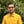Trusted answers to developer questions
Trusted Answers to Developer Questions

Related Tags

d
communitycreator

# What is log() in D?Harris Amjad

Grokking Modern System Design Interview for Engineers & Managers

Ace your System Design Interview and take your career to the next level. Learn to handle the design of applications like Netflix, Quora, Facebook, Uber, and many more in a 45-min interview. Learn the RESHADED framework for architecting web-scale applications by determining requirements, constraints, and assumptions before diving into a step-by-step design process.

### Overview

The log() function in D calculates the natural logarithm of a number.

The image below shows the mathematical representation of the log() function:

Mathematical representation of the log() function

Note: Import std.math in your code to use the log() function. We can import it like this:

import std.math


### Syntax

Following is the syntax for the method:

log(number)
// number should be float, double or real.


### Parameter

This function requires a numbermust be greater than 0 for which the logarithm is to be calculated.

### Return value

log() returns the natural logarithm of the number sent as a parameter.

Note:

• If the parameter value is positive infinity, then log() returns positive infinity.
• If the parameter value is NaN, or negative infinity, then log() returns NaN.
• If the parameter value is -NaN, then log() returns -NaN.
• If the parameter value is <= 0, then log() returns negative infinity.

### Example

The code below shows the use of the log() function in D:

import core.stdc.stdio;import std.stdio;//header required for functionimport std.math;int main(){    //integer    writeln ("The value of log(4.0) : ",log(4.0));    //positive double value    writeln ("The value of log(2.56) : ",log(2.56));    //e    writeln ("The value of log(E) : ",log(E));    //exceptional outputs    writeln ("The value of log(-0.0) : ",log(-0.0));    writeln ("The value of log(0.0) : ",log(0.0));    writeln ("The value of log(real.infinity) : ",log(real.infinity));    writeln ("The value of log(-real.infinity) : ",log(-real.infinity));    writeln ("The value of log(real.nan) : ",log(real.nan));    writeln ("The value of log(-real.nan) : ",log(-real.nan));    return 0;}

### Explanation

• Line 4: We add the header std.math required for log() function.

• Line 9: We calculate the natural log of the integer value using log().

• Line 12: We calculate the natural log of the positive double value using log().

• Line 15: We calculate the natural log of the e using log().

• Line 18: We calculate the natural log of exceptional numbers using log().

RELATED TAGS

d
communitycreator

CONTRIBUTORHarris Amjad

Grokking Modern System Design Interview for Engineers & Managers

Ace your System Design Interview and take your career to the next level. Learn to handle the design of applications like Netflix, Quora, Facebook, Uber, and many more in a 45-min interview. Learn the RESHADED framework for architecting web-scale applications by determining requirements, constraints, and assumptions before diving into a step-by-step design process.

Keep Exploring

Learn in-demand tech skills in half the time